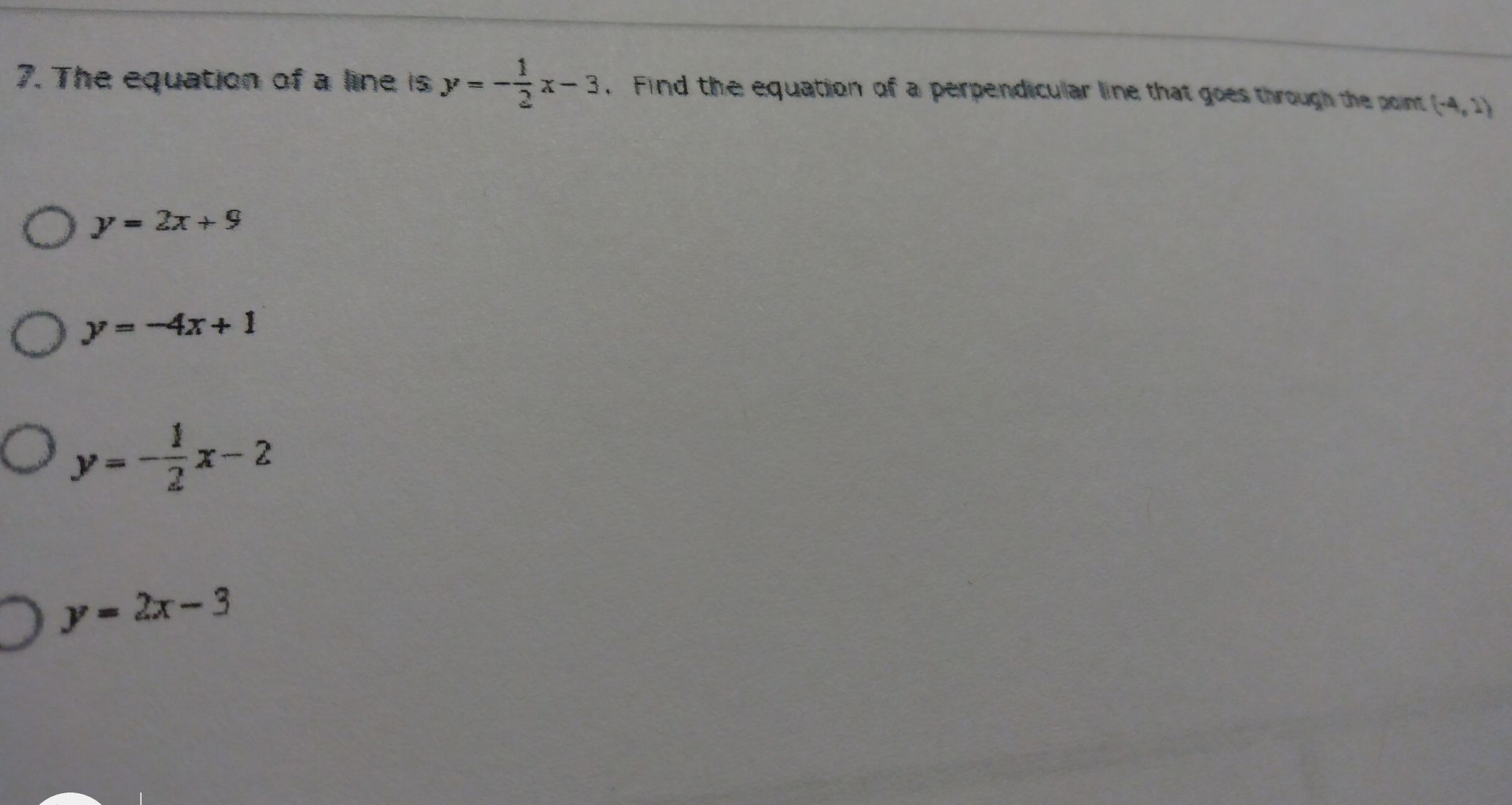### ¿Todavía tienes preguntas de matemáticas?

Pregunte a nuestros tutores expertos
Algebra
Pregunta7. The equation of a fine is $$y = - \frac { 1 } { 2 } x - 3$$ . Find the equation af a perpendicular line that goes through the $$x a m ( - 4,2 )$$

$$y = 2 x + 9$$

$$y = - 4 x + 1$$

$$y = - \frac { 1 } { 2 } x - 2$$

$$y = 2 x - 3$$

$$y = 1$$

$$y= 2x- 3$$